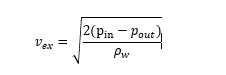Bernoulli's Equation and water rockets

So i'm playing around with some water rockets and I'm trying to figure out how fast the exhaust velocity of the water is. I've had an experimental approach using high fps camera to record and analyse (using tracker) the exhaust velocity. I'm using a 0,5 l soda bottle with 0,085 L ; 0,135 ; L 0,185 L and 0,235 L water. So far i've found, that more water means less exhaust velocity (the pressure is exactly the same at 1,8 bar). This makes sense, because the acceleration of the water comes from the energy of the pressurized air.
However i've found, that alot of people use the Bernoullis equation (rewritten) to calculate the exhaust velocity with the formula:However there is no mass involved here?

The denominator is density. Mass is 'involved' there.

•vanhees71 and russ_watters
The denominator is density. Mass is 'involved' there.
Indeed, but then it doesn't matter if there is 50 ml of water or 450 ml of water. This makes no sense to me tbh.

It doesn't matter, for the velocity calculation.
I suspect that the reason that more water results in lower velocity is because there is less gas. The pressure decays as the water is expelled - that rate of reduction is higher if there is less gas (initially). The initial velocities should be the same.

•Ahh, thanks! That makes perfectly sense!
I was wondering if there is a good model for pressure as a function of time?

russ_watters
Mentor
Indeed, but then it doesn't matter if there is 50 ml of water or 450 ml of water. This makes no sense to me tbh.
If you have density and you want mass, you just multiply by volume. If you have velocity and you want volumetric flow rate, multiply by cross sectional area. Like any equation/model, Bernoulli's equation is adaptable, you just have to ask it the question you want it to answer!

•sophiecentaur, vanhees71 and Bystander
Mister T
Gold Member
Indeed, but then it doesn't matter if there is 50 ml of water or 450 ml of water. This makes no sense to me tbh.

If you have nine times as much volume you also have nine times as much mass. Think of it this way. When 450 mL of water is expelled you can imagine breaking that stream of ejected fluid into nine equal 50 mL segments. The last segment, for example, is ejected at the same speed as the first segment, provided the pressure difference is the same.

gmax137
provided the pressure difference is the same.
Yes, but this is not the situation for a 1/2 liter bottle initially filled halfway with liquid. The gas pressure (driving the flow out the nozzle) decreases as the liquid is discharged and the gas expands.

You can use Bernoulli to determine the initial nozzle velocity (pretty much pressure head becomes velocity head) but to solve for the time-dependent velocity you have to also model the expansion of the gas.

cjl
For the gas expansion, I'd just model it as adiabatic expansion, so for air, p*v^1.4 = constant. You can get dv/dt from the exhaust velocity and nozzle diameter, so from that, you should be able to figure out the pressure (and velocity) at all points during the expulsion of the water.

•MigMRF
For the gas expansion, I'd just model it as adiabatic expansion, so for air, p*v^1.4 = constant. You can get dv/dt from the exhaust velocity and nozzle diameter, so from that, you should be able to figure out the pressure (and velocity) at all points during the expulsion of the water.
Yeah, that might actually be a solution. Just to make sure, when you write dv/dt are you saying v as velocity or do you mean V as in volume. It would make more sense if the change in volume with respect to time is related to the mass flow rate

Mister T
Gold Member
Yes, but this is not the situation for a 1/2 liter bottle initially filled halfway with liquid.

You introduced the equation ##v_{ex}=\sqrt{\frac{2(p_{in}-p_{out})}{\rho_w}}## into this discussion, and wanted to understand the role played by mass. I tried to explain that to you.

The fact that that ##p_{in}## is not constant in your particular application of that equation has no bearing on the explanation. In other words, try to understand the role played by mass when ##p_{in}## is constant so that you can then understand the more complicated situation when it's not constant.

cjl